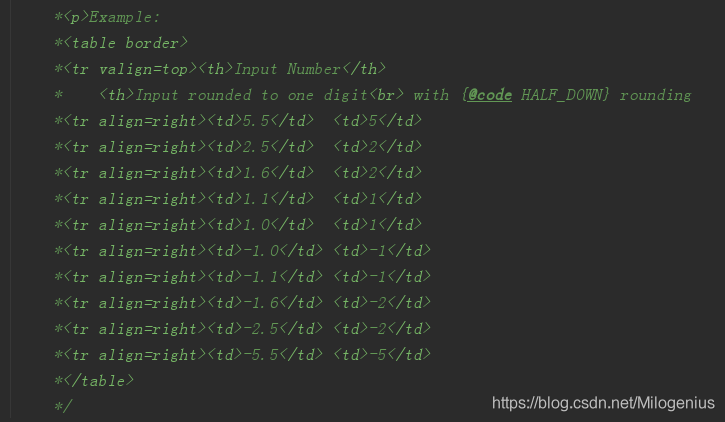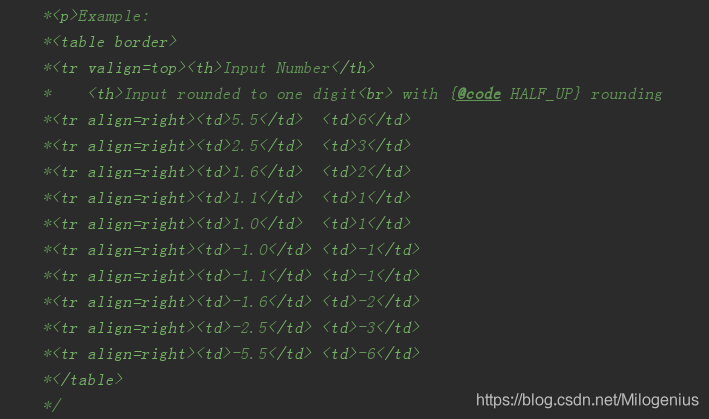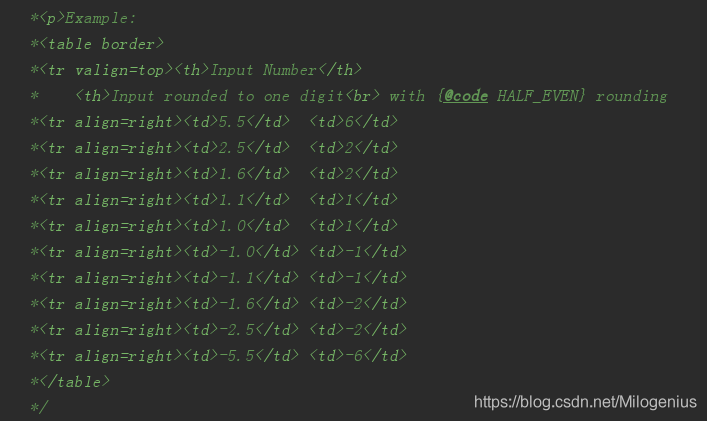Java中的浮点数四舍五入到小数点后2位的几种方法麦洛1393 0 0

## 相关知识点

### 1.Java中的舍入模式

`RoundingMode.HALF_DOWN`：如果两个邻居等距，则向下舍入，例如2.5将舍入为2.0`RoundingMode.HALF_UP`：如果两个邻居的距离相同则向上舍入，例如2.5将舍入为3.0`RoundingMode.HALF_EVEN`：如果两个邻居等距，则向偶数舍入，例如2.5将舍入到2.0，而5.5将舍入到6.0### 2.使用BigDecimal将数字四舍五入到小数点后两位

``````package com.milo.base.sort;

import org.junit.Test;

import java.math.BigDecimal;

/**
* 测试BigDecimal的Api
* @author milogenius
* @date 2020-04-14 19:49
*/
public class BigDecimalTest {

@Test
public void test1(){
float number = BigDecimal.valueOf(4.526f)
.setScale(2, BigDecimal.ROUND_HALF_DOWN)
.floatValue();
System.out.println(number);
}
}
----------------------------------------------
Output:
4.53``````

`setScale（）`中，您可以指定需要舍入的小数位数，在这里，我们将小数位数设置为2，因为我们将舍入到2位数字。 我们指定舍入模式`ROUND_HALF_DOWN`，类似于`RoundingMode.HALF_DOWN`。 您也可以使用`RoundingMode.HALF_DOWN`来代替`BigDecimal.ROUND_HALF_DOWN`，但是要注意，从Java 5开始添加了`RoundingMode`枚举。

### 3.使用DecimalFormat将数字四舍五入到小数点后两位

``````    @Test
public void test2(){
DecimalFormat df = new DecimalFormat("#.00");
float number = Float.valueOf(df.format(4.526f));
System.out.println(number);
}

----------------------------------------------
Output:
4.53
``````

`＃.00`用于四舍五入到小数点后两位，如果要四舍五入到小数点后第三位，只需使用`＃.000``＃.0000`来创建`DecimalFormat`。 要了解有关格式化数字的更多信息，请参见如何使用`DecimalFormat`在Java中格式化数字。

### 4.使用Math.round（）在Java中进行舍入运算

`Math.round（）`是Java中舍入数字的经典方法。 尽管它没有提供任何`API`来指定要舍入的位数，但是通过使用乘法和除法，您可以将其舍入到n或2个小数位。

``````    @Test
public void test3(){
float rounded = (float) Math.round(4.526f*100)/100;
System.out.println(rounded);
}
----------------------------------------------
Output:
4.53``````

## 综合案例

``````package com.milo.base.sort;

import java.math.BigDecimal;
import java.text.DecimalFormat;
import java.util.Arrays;

/**
* 使用三种方式演示java中保留2位小数
* 包括 BigDecimal,
* Math.round and DecimalFormat class.
* @author milogenius
*/

public class RoundingNumbers {

public static void main(String args[]) {

//数据
float[] samples = new float[]{2.123f, 2.125f, 2.127f};

//使用 Match.round()保留2位小数
float[] rounded = round(samples);

System.out.println("开始舍入, numbers: "
+ Arrays.toString(samples));
System.out.println("结束舍入 : 使用Match.round()方法 "
+ Arrays.toString(rounded));

//使用BigDecimal保留2位小数
//我们可以使用不同的舍入模式e.g., HALF_UP, HALF_DOWN,and HALF_EVEN with BigDecimal
rounded = roundUsingBigDecimal(samples);

System.out.println("开始舍入,numbers : "
+ Arrays.toString(samples));

System.out.println("结束舍入, number 使用 BigDecimal: "
+ Arrays.toString(rounded));

//DecimalFormat Example to round number to 2 digits
//使用DecimalFormat来保留2位小数
rounded = roundUsingDecimalFormat(samples);
System.out.println("开始舍入numbers: "
+ Arrays.toString(samples));
System.out.println("结束舍入,使用DecimalFormat: "
+ Arrays.toString(rounded));

}

/**
*  使用 Math.round保留2为小数
* @param numbers
* @return
*/
public static float[] round(float[] numbers) {

float[] round = new float[numbers.length];
for (int i = 0; i < numbers.length; i++) {
float number = numbers[i];
round[i] = (float) Math.round(number * 100) / 100;
}
return round;
}

/**
* 使用BigDecimal保留2为小数
* @param digits
* @return
*/
public static float[] roundUsingBigDecimal(float[] digits) {
float[] result = new float[digits.length];
for (int i = 0; i < digits.length; i++) {
float digit = digits[i];
result[i] = BigDecimal.valueOf(digit)
.setScale(2, BigDecimal.ROUND_HALF_DOWN)
.floatValue();
}
return result;
}

/**
* 使用DecimalFormat保留2为小数
* @param decimals
* @return
*/
public static float[] roundUsingDecimalFormat(float[] decimals) {
float[] rounded = new float[decimals.length];
//DecimalFormat to round numbers to 2 decimal place
DecimalFormat df = new DecimalFormat("#.00");
for (int i = 0; i < decimals.length; i++) {
float decimal = decimals[i];
rounded[i] = Float.valueOf(df.format(decimal));
}
return rounded;
}
}
``````

## 总结

### 相关推荐

Java中的浮点数四舍五入到小数点后2位的几种方法

Java BigDecimal使用
1.引言 ----  float和double类型的主要设计目标是为了科学计算和工程计算。他们执行二进制浮点运算，这是为了在广域数值范围上提供较为精确的快速近似计算而精心设计的。然而，它们没有提供完全精确的结果，所以不应该被用于要求精确结果的场合。但是，商业计算往往要求结果精确，这时候BigDecimal就派上大用场啦。 测试用例： publi
Java 的BigDecimal

Java中BigDecimal的8种舍入模式
java.math.BigDecimal ==================== 不可变的、任意精度的有符号十进制数。BigDecimal 由任意精度的整数非标度值和32位的整数标度(scale)组成。 如果为零或正数，则标度是小数点后的位数。如果为负数，则将该数的非标度值乘以10的负scale次幂。 因此，BigDecimal表示的数值是(unsc
Java中的BigDecimal类和int和Integer总结
### 前言 我们都知道浮点型变量在进行计算的时候会出现丢失精度的问题。如下一段代码： System.out.println(0.05 + 0.01); System.out.println(1.0 - 0.42); System.out.println(4.015 * 100); System.out.println(1
Java的BigDecimal容易出现的坑
BigDecimal一般是用来做要求比较高的精准计算的。前几天在使用的时候遇到一个大坑，记录下。 这个问题产生是使用BigDecimal做除法(divide)运算，这个类的divide方法存在三个常用的构造函数。 `BigDecimal` `**divide**(BigDecimal divisor)`            Returns a `
Java：利用BigDecimal类巧妙处理Double类型精度丢失

java 大数基本操作

java 泛型中遇到的问题

java.math.BigDecimal类的用法

java中经常问到的面试题
1、面向对象编程的三大特性是什么? ================= (1).继承： 继承是一种联结类的层次模型，并且允许和鼓励类的重用，它提供了一种明确表述共性的方法。对象的一个新类可以从现有的类中派生，这个过程称为类继承。新类继 承了原始类的特性，新类称为原始类的派生类（子类），而原始类称为新类的基类（父类）。派生类可以从它的基类那里继承方法和实例
java浮点型精度丢失浅析
java浮点型数值在运算中会出现精度损失的情况，在业务要求比较高比如交易等场景，一般使用BigDecimal来解决精度丢失的情况。最近一个同事在使用BigDecimal时仍然出现了精度损失，简略记录一下 ### 测试用例 代码如下 @Test public void fd() { double abc =
BigDecimal与Long、int之间的相互转换

BigDecimal去除末尾多余的0
Java有自带的 stripTrailingZeros() 方法用于去除末尾多余的0 BigDecimal num = new BigDecimal("100.000"); BigDecimal numNoEndZero = num.stripTrailingZeros(); //numNoEndZero ：1E+2 Sys
BigDecimal的四则运算及小数位数格式

HelloWorld开发者社区 - 开发者专属的技术社区